LinkageMapView是一个用R编写的免费的包，可以绘制遗传连锁图谱和QTL图谱，我们在拿到遗传图谱之后，可以通过此功能来对图谱进行可视化，展示的形式也是多种多样的，还可以在图片上增加QTL的内容，最方便的是可以直接将R/qtl的输出作为LinkageMapView的输入，也可以接收txt和csv格式。

#### 1.下面具体介绍一下包的安装和使用

```Install.packages(“LinkageMapView”) ```

#### ```library(LinkageMapView) ```

LinkageMapView包中主要有一个函数——lmv.linkage.plot，包括的参数非常多，在说明文档中仅仅是参数说明就用了4页，所以我们了解一些常用的即可。

2.下面进行实例介绍

1、最简单的图谱绘制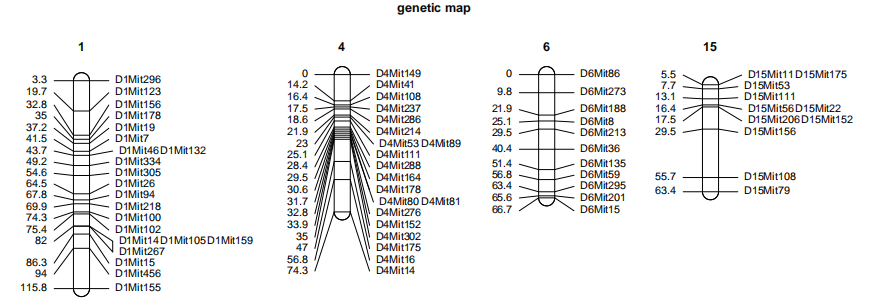library(qtl)
data(hyper)
lmv.linkage.plot(mapthis=hyper,outfile=”a.pdf”,mapthese=c(1,4,6,15),main=”genetic map”)

#### 2、变更部分标记名称颜色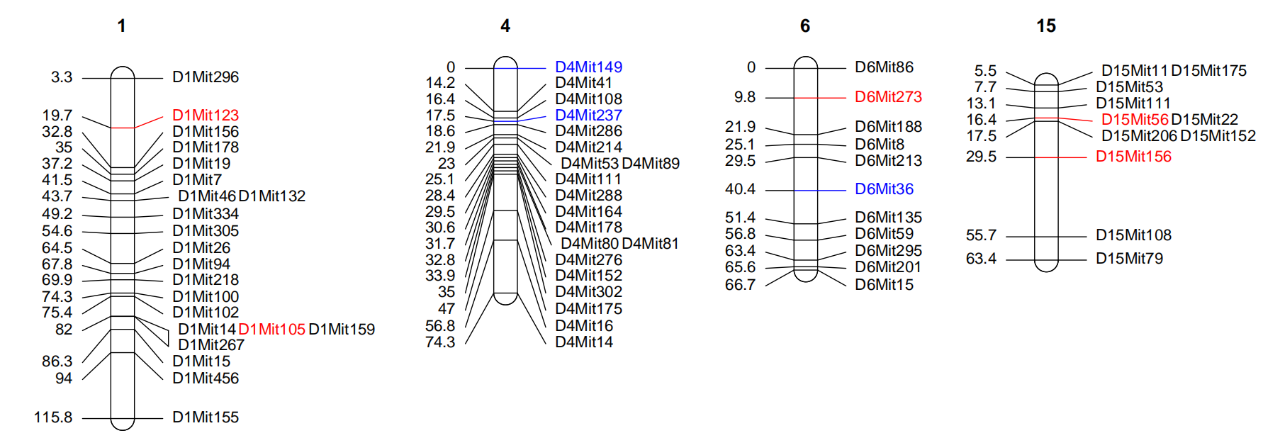flist[] <- list(locus = c(“D1Mit123″,”D1Mit105″,”D6Mit273″,”D15Mit56″,”D15Mit156”),col=c(“red”))
flist[] <- list(locus = c(“D4Mit149″,”D4Mit237″,”D6Mit36”),col=c(“blue”))
lmv.linkage.plot(hyper,”b.pdf”,mapthese=c(1,4,6,15),markerformatlist=flist)

#### 3、颜色设置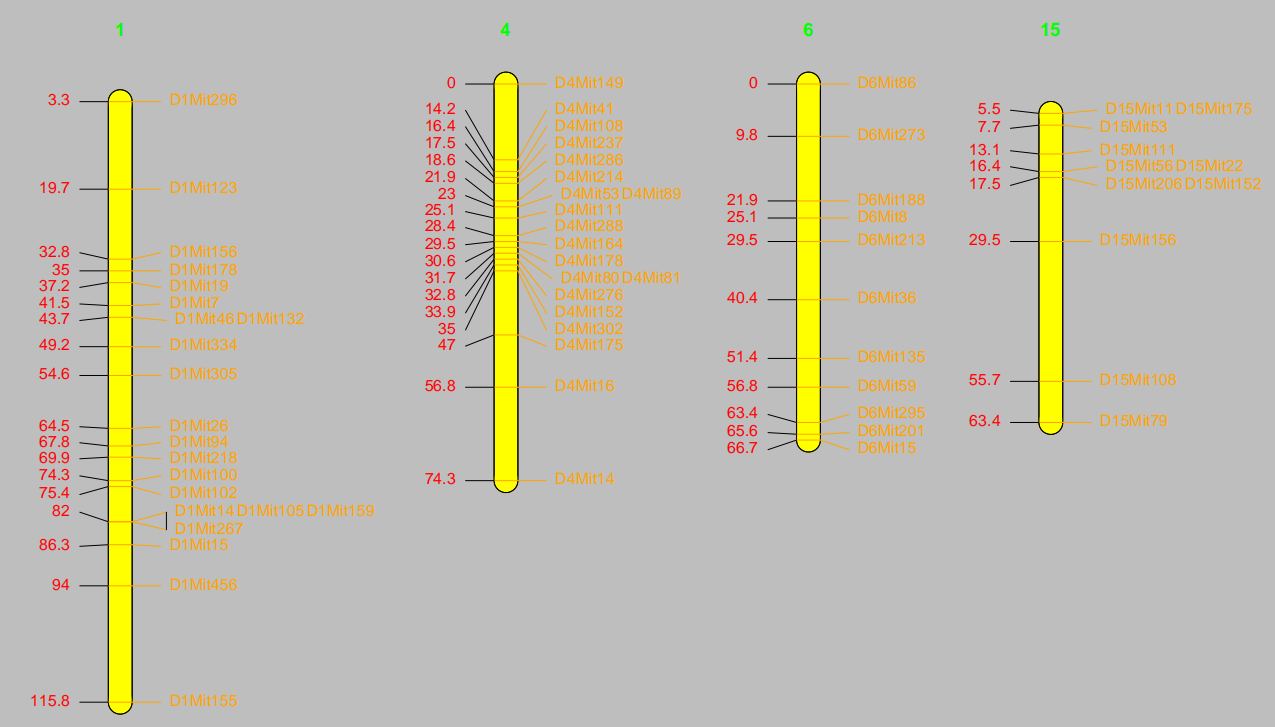lmv.linkage.plot(hyper,”c.pdf”,
mapthese=c(1,4,6,15),
pdf.bg=”grey”,col.lgtitle=”green”,
lg.col=”yellow”,lcol=”red”,rcol=”orange”)

pdf.bg：pdf背景色
col.lgtitle：连锁群名称颜色
lg.col：连锁群柱子颜色
lcol：遗传位置label的颜色
Rcol：标记名称label的颜色

4、字体、字号设置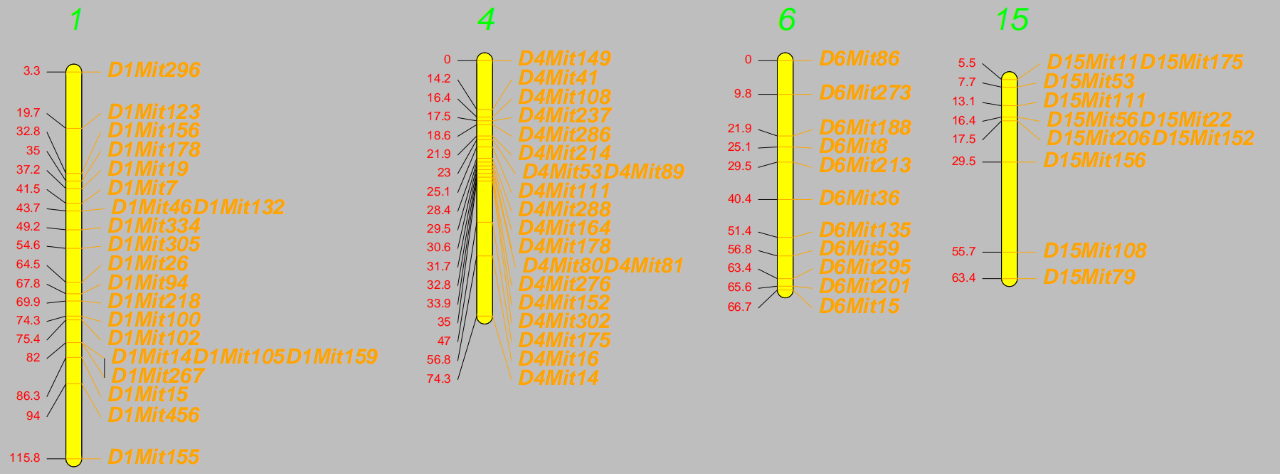lmv.linkage.plot(hyper,”d.pdf”,mapthese=c(1,4,6,15),pdf.bg=”grey”,col.lgtitle=”green”,               pdf.height=8,lg.col=”yellow”,lcol=”red”,rcol=”orange”,                 lfont=1,lcex=1.2,rfont=4,rcex=2,font.lgtitle=3,cex.lgtitle=3)

lfont：遗传位置label的字体设置，1代表纯文本，2代表粗体，3代表斜体，4代表粗斜体
lcex:遗传位置label的字号大小设置
rfont：标签label的字体设置
rcex：标签label的字号大小设置
font.lgtitle：连锁群编号字体设置
cex.lgtitle：连锁群编号字号大小设置

5、部分染色体柱子上色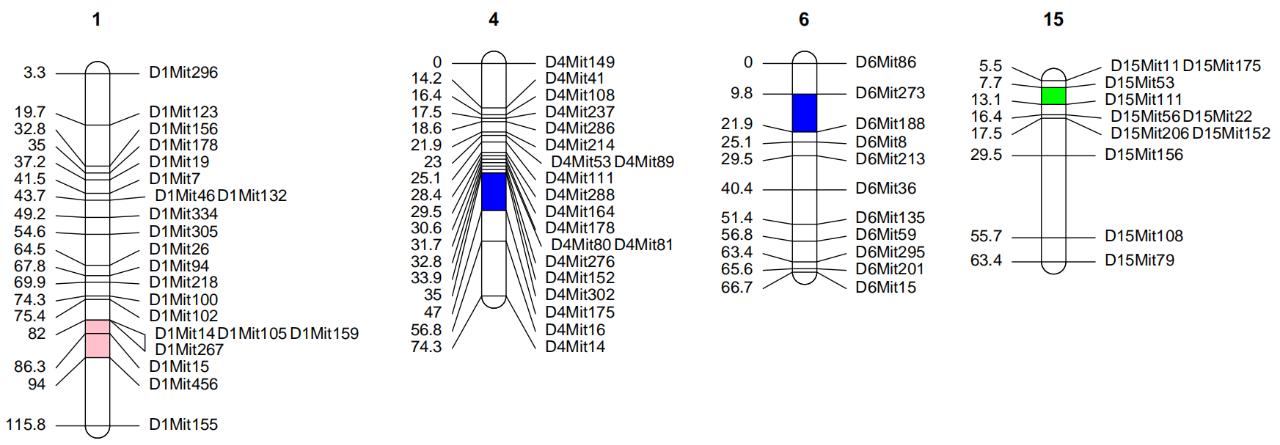chr = c(1, 4, 6, 15)
s = c(82,35,9.8,7.7)
e = c(94,47,21.9,13.1)
col = c(“pink”,”blue”,”blue”,”green”)
sectcoldf <- data.frame(chr, s, e, col,stringsAsFactors = FALSE)
lmv.linkage.plot(hyper,”e.pdf”,mapthese=c(1,4,6,15),             sectcoldf=sectcoldf)

sectcoldf为连锁群柱子部分上色参数，上色信息以data.frame格式储存，每一行是一个上色区域，第一列是连锁群编号，第二列是对应连锁群上色起始，第三列是上色终止，第四列是对应的颜色。

6、标签显示形式设置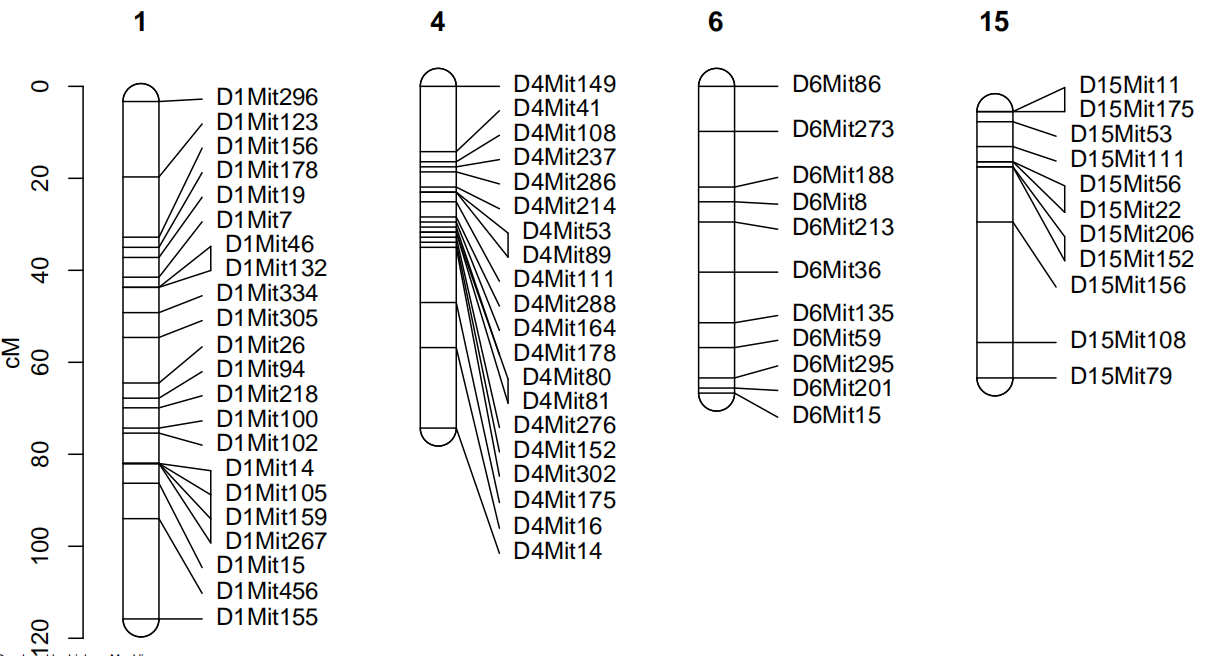ruler=TRUE代表用纵坐标表示遗传位置，FALSE代表以位置label的形式表示遗传位置，默认FALSE。

Maxnbrcolsfordups 表示标记label用几列表示，默认3.

7、相同标记名称连线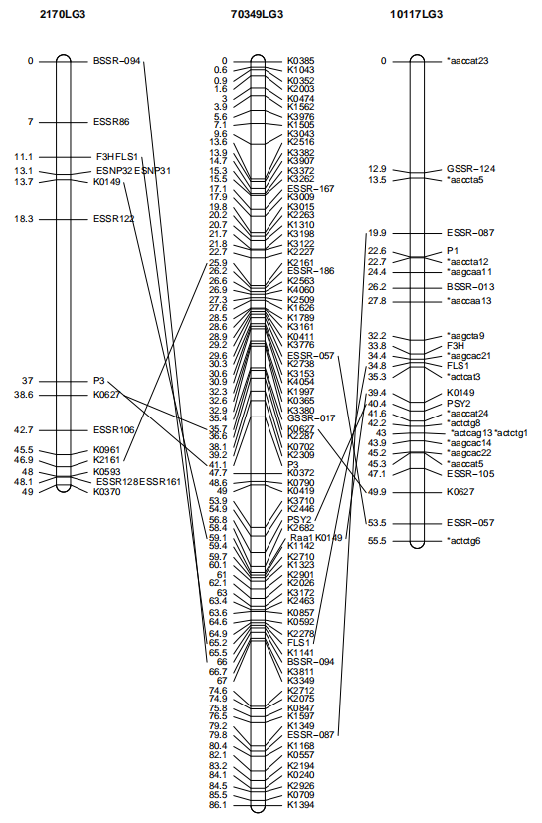data(carrot)
lmv.linkage.plot(mapthis = carrot,outfile = “g.pdf”)

8、标记名称不同连线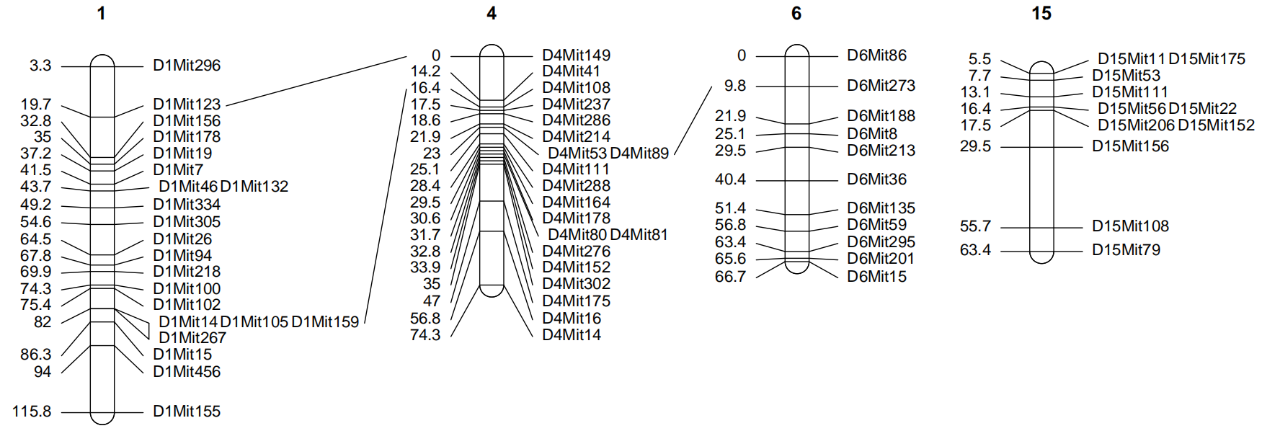```fromchr = c(1,1,4) fromlocus = c("D1Mit123","D1Mit105","D4Mit53") tochr = c(4,4,6) tolocus = c("D4Mit149","D4Mit108","D6Mit273") conndf <- data.frame(fromchr,fromlocus,tochr,tolocus,stringsAsFactors = FALSE) lmv.linkage.plot(hyper,"h.pdf",mapthese = c(1,4,6,15),conndf = conndf)```需要连线的标记对以data.frame存储，每行一对连线，第一列是线一端的起始连锁群编号，第二列是起始的标记名称，第三列是线另一端终止连锁群编号，第四列是终止的标记名称。

9、翻转连锁群顺序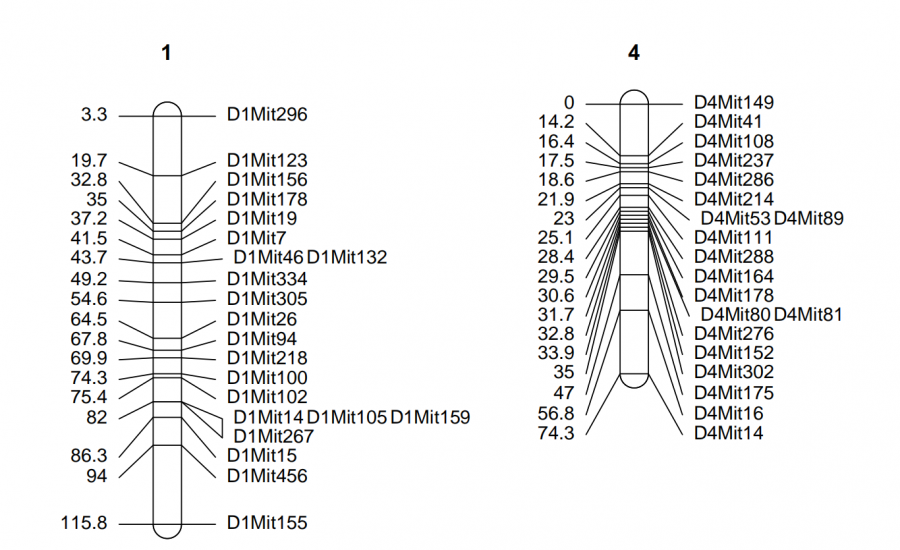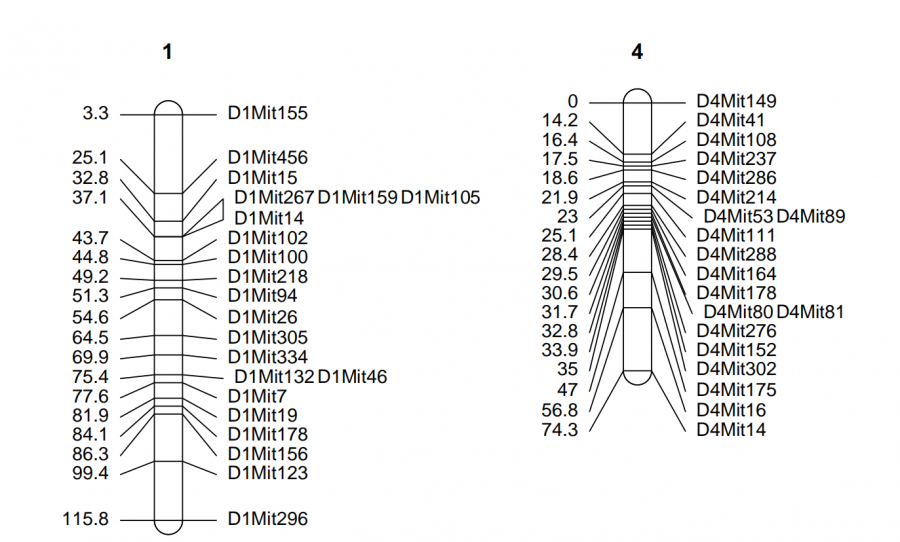lmv.linkage.plot(hyper,”i.pdf”,mapthese = c(1,4),revthese = “1”)

revthese参数后面接需要翻转的连锁群编号

10、密度图谱绘制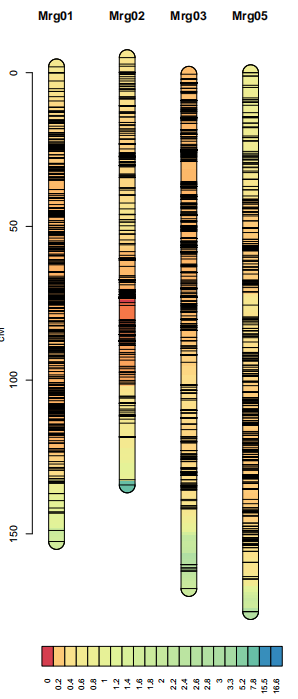data(oat)
lmv.linkage.plot(oat,”j.pdf”,mapthese=c(“Mrg01″,”Mrg02″,”Mrg03″,”Mrg05”),denmap=TRUE)

denmap是控制是否画密度图的开关，画密度图的同时不显示标记名称和遗传位置信息。

11、QTL绘制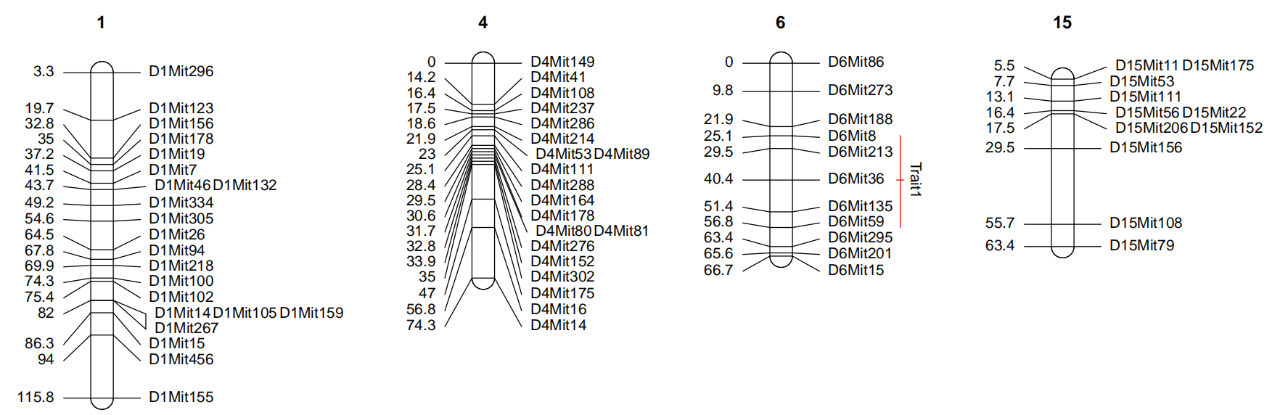qtldf <- data.frame(
chr = character(),
qtl = character(),
so = numeric(),
si = numeric(),
ei = numeric(),
eo = numeric(),
col = character(),
stringsAsFactors = FALSE
)
qtldf <- rbind(qtldf,
data.frame(
chr = “6”,
qtl = “Trait1″,
so = 25,
si = 40.4,
ei = 40.4,
eo = 56.8,
col=”red”
))
lmv.linkage.plot(hyper,”k.pdf”, mapthese=c(1,4,6,15),qtldf = qtldf)

qtldf参数后接qtl信息，qtl信息存储在data.frame格式的qtldf里，一行一个qtl信息，第一列是连锁群编号，第二列是qtl名，第三列是qtl外区间起始，第四列是qtl内区间起始，第五列是qtl内区间终止，第六列是qtl外区间终止，第七列是颜色。